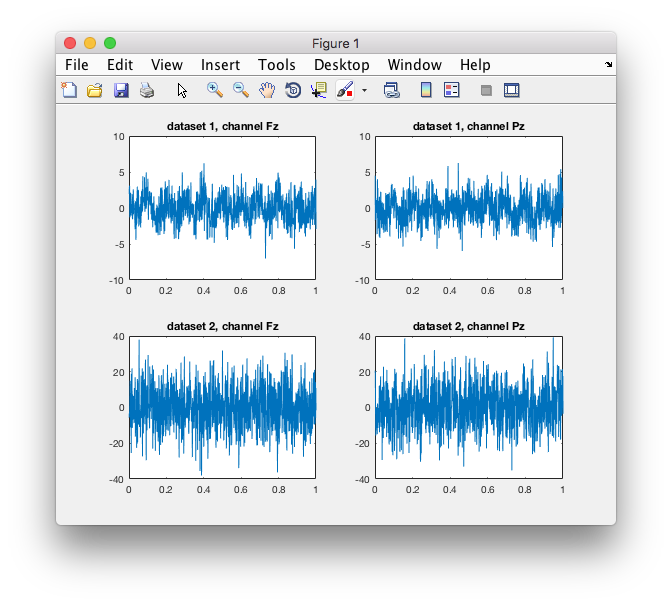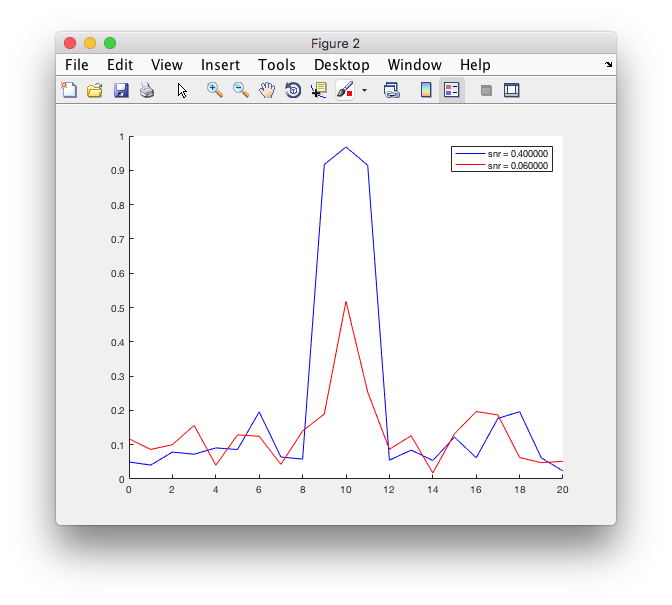Tags: example freq coherence

# Effect of SNR on Coherence

This example script uses simulated signals to demonstrate the effect of the signal to noise ratio (SNR) on the coherence estimate. Two datasets are simulated that have the same underlying signal, but that have different amounts of noise.

Note that in this example we are changing the noise. We could also have kept the noise constant and have varied the amplitude of the signal. The results of the simulated would not be different.

Specify the parameters of the two datasets.

``````fsample   = 1000;
fsignal   = 10;
nsamples  = 1000;
taper     = 'hanning';

% spectral leakage can be investigated with these parameters
% fsignal   = 10.5;
% taper     = 'boxcar';

% you could explore the effect of different numbers of trials
ntrials1   = 100;
ntrials2   = 100;

% introduce a random phase difference between the two channels on each trial
phaseFz = 2*pi * (zeros(1,ntrials)                      );
phasePz = 2*pi * (zeros(1,ntrials) + 0.1*rand(1,ntrials));

% the two datasets have the same signal, but a different amount of noise
snr1 = 0.400;
snr2 = 0.060;
``````

Compute two simulated datasets, each with the same channels.

``````data1 = [];
data1.label = {'Fz', 'Pz'};
for i=1:ntrials1
data1.time{i} = (1:nsamples)/fsample;
data1.trial{i} = [
cos(fsignal * 2*pi * data1.time{i} + phaseFz(i)) + (1/snr1) * randn(1,nsamples)/sqrt(2);
cos(fsignal * 2*pi * data1.time{i} + phasePz(i)) + (1/snr1) * randn(1,nsamples)/sqrt(2);
];
end

data2 = [];
data2.label = {'Fz', 'Pz'};
for i=1:ntrials2
data2.time{i} = (1:nsamples)/fsample;
data2.trial{i} = [
cos(fsignal * 2*pi * data2.time{i} + phaseFz(i)) + (1/snr2) * randn(1,nsamples)/sqrt(2);
cos(fsignal * 2*pi * data2.time{i} + phasePz(i)) + (1/snr2) * randn(1,nsamples)/sqrt(2);
];
end

figure
% note that all subplots will have individual scaling
subplot(2,2,1); plot(data1.time{1}, data1.trial{1}(1,:)); title(sprintf('dataset 1, channel %s', data1.label{1}));
subplot(2,2,2); plot(data1.time{1}, data1.trial{1}(2,:)); title(sprintf('dataset 1, channel %s', data1.label{2}));
subplot(2,2,3); plot(data2.time{1}, data2.trial{1}(1,:)); title(sprintf('dataset 2, channel %s', data2.label{1}));
subplot(2,2,4); plot(data2.time{1}, data2.trial{1}(2,:)); title(sprintf('dataset 2, channel %s', data2.label{2}));
``````Next we compute the spectral decomposition of the raw data and subsequently compute the coherence with ft_connectivityanalysis. Note that for coherence (or other measures of phase synchrony) we need to specify either ‘powandcsd’ or ‘fourier’ as cfg.output to ft_freqanalysis.

``````cfg = [];
cfg.method = 'mtmfft';
cfg.taper = taper;
cfg.output = 'powandcsd';
cfg.foilim = [0 2*fsignal];
freq1 = ft_freqanalysis(cfg, data1);
freq2 = ft_freqanalysis(cfg, data2);

cfg = [];
cfg.method = 'coh';
conn1 = ft_connectivityanalysis(cfg, freq1);
conn2 = ft_connectivityanalysis(cfg, freq2);

figure
hold on
plot(conn1.freq, conn1.cohspctrm, 'b');
plot(conn2.freq, conn2.cohspctrm, 'r');
legend({sprintf('snr = %f', snr1), sprintf('snr = %f', snr2)});
``````Plotting the coherence for the two datasets is easy, as there are only two channels and therefore one estimate of coherence (as function of frequency) per dataset. For more realistic numbers of channels you may want to look at ft_connectivityplot and ft_multiplotCC.

## Exercise 1

You should vary the SNR parameters for the two datasets and experiment with the amount of random phase difference between the Fz and Pz channel.

## Exercise 2

You should set the frequency of the signal either at 10 Hz or at 10.5 Hz and experiment with the taper in ft_freqanalysis. Especially for large signal to noise values you will see that the spectral leakage for a signal at 10.5 Hz is much larger with a boxcar taper than with a tanning taper.

## Exercise 3

You should make the SNR the same between conditions, but vary the number of trials.

## Exercise 4

You should change the code such that the noise amplitude is constant, but that the signal amplitude depends on the SNR ratio.